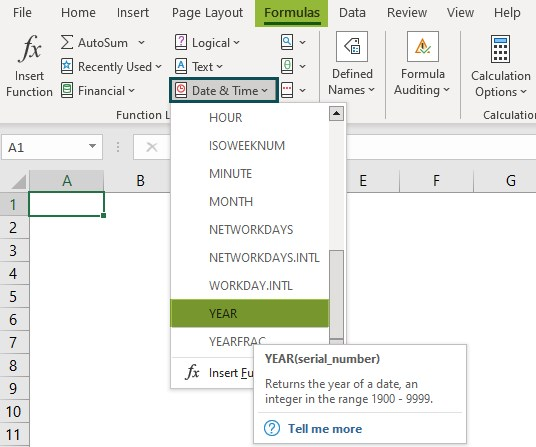# YEAR Function In ExcelArticle byExcelMojo Team## What Is YEAR Function In Excel?

The YEAR function in Excel extracts the corresponding years of the selected dates or date value cells. The function will always return a 4-digit year, ranging from 1900 to 9999. The function also provides the year value to another formula.

The YEAR function in Excel is an inbuilt function, so that we can insert the formula from the “Functional Library” or enter it directly in the worksheet.

For example, we will apply the YEAR function to calculate the year from the given date value. Select cell B2, enter the formula =YEAR(A2), and press the “Enter” key.

The output is “2022”, as shown above.

###### Key Takeaways
• The YEAR function in Excel is a “Date & Time” function that retrieves the year from a specific date. The function returns a 4-digit number output.
• We can use the YEAR formula along with the DATE() and TODAY() functions.
• The input dates supplied in the YEAR function in Excel should be serial numbers or references to cells with valid dates or valid date values.
• If we give any integer value from 1 to 31 as input, by default, Excel returns the year as 1900. If the number is above 31, Excel counts the 365 days per year and displays the year of that particular serial_number year.

### YEAR() Excel Formula

The Syntax of the YEAR Excel Formula is,

The argument of the YEAR Excel Formula is,

• serial_number: It is a mandatory number. It is given to extract the Year from the specified date or serial number. The return value will always be between 1900 and 9999.

[Note: The number 1 serial number is for the date 1-1-1900. Hence, the range 1900 to 9999 is followed.]

### How To Use The YEAR Excel Function?

We can use the YEAR function in Excel in 2 methods, namely,

1. Access from the Excel ribbon.
2. Enter in the worksheet manually.

#### #1 Method – Access from the Excel ribbon

First, choose an empty cell – select the “Formulas” tab – go to the “Function Library” group – click the “Date & Time” option drop-down – select the “YEAR” function, as shown below.

The “Function Arguments” window appears. Enter the argument values, in the serial_number field -> click “OK”, as shown below.

#### #2 Method – Enter in the worksheet manually

1. Select an empty cell for the output.
2. Type =YEAR( in the selected cell. Alternatively, type “=Y” and double-click the YEAR function from the list of suggestions shown by Excel.]
3. Enter the argument as a value or a cell reference in Excel and close brackets.
4. Press the “Enter” key.

#Basic Example

We will extract the years from the values using YEAR function in Excel.

In the table, the data is,

• Column A contains the Date.

The steps to extract the year using YEAR function in Excel are as follows:

1. Select cell B2 and enter the formula =YEAR(A2) [value of ‘serial_number’ as “A2”.]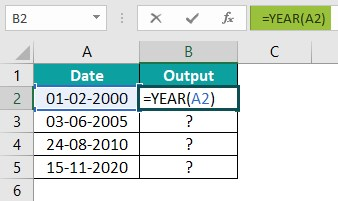2. Press the “Enter” key. The result is “2000”, as shown below.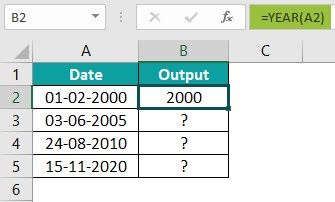3. Drag the formula from cell B2 to B5 using the fill handle. The output is shown below.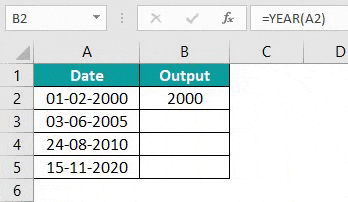### Examples

We will understand the YEAR function in Excel examples with advanced scenarios.

#### #1 – Example

We will extract the years from the values using the YEAR function.

In the table, the data is,

• Column A contains the Date.
• Column B displays the Output.

The steps to extract the year using the YEAR function in Excel are as follows:

Step 1: Select cell B2, enter the formula =YEAR(A2), and press the “Enter” key. The result is “1934”, as shown below.

Step 2: Drag the formula from cell B2 to B5 using the fill handle. The output is shown below.

#### #2 – Example

We will extract the year from the values using the YEAR function in Excel.

In the table, the data is,

• Column A contains the Date.
• Column B displays the Output.

The steps to extract the year values using the YEAR function in Excel are as follows:

Step 1: Select cell B2, enter the formula =YEAR(A2), and press the “Enter” key. The result is “1900”, as shown below.

Step 2: Drag the formula from cell B2 to B5 using the fill handle. The output is shown below.

#### #3 – Example

We will extract the year from the values using the YEAR function and the TODAY Excel function.

In the table, the data is,

• Column A displays the Output.

The steps to extract the years using the TODAY() and the YEAR() functions are as follows:

Step 1: Select cell A2 and enter the formula =YEAR(TODAY()). [TODAY() is the serial_number value.]

Step 2: Press the “Enter” key.

The result is “2022”, as shown above. First, the Today() function calculates the current date, and then the year is extracted and displayed as output.

#### #4 Example

We will extract the year using the YEAR function and the DATE excel function.

The steps to extract the year using the YEAR() and the DATE() functions are as follows:

In the table, the data is,

• Column A displays the Output.

Step 1: Select cell A2, enter the formula =YEAR(DATE(2011,8,26)). [ DATE(2011,8,26) is the serial_number value.]

Step 2: Press the “Enter” key. The result is “2011”, as shown below.

### Important Things To Note

• We get the VALUE!” error, if the dates provided are less than 1900 or greater than 9999.
• If the specified date value or cell is missing either the date, month, or year.
• The YEAR function accurately extracts the years, irrespective of the input date formats in Excel.
• The format of the YEAR function should be general or number, or it will show the result as a date.

1. What does the YEAR function do in Excel?

The YEAR function in Excel calculates the serial number or the year from a given date. The function takes the date in any date format as an input and returns a four-digit numeric value as an integer value year of the specified date.

The syntax of the YEAR function is =YEAR(serial_number)

2. How does the YEAR function work in Excel?

We can work with the YEAR function in Excel as follows:

#1 – Select an empty cell for the output.
#2 – Type =YEAR( in the selected cell. Alternatively, type “=Y” and double-click the YEAR function from the list of suggestions shown by Excel.]
#3 – Enter the argument as a value or a cell reference and close brackets.
#4 – Press the “Enter” key.

For example, the below image depicts the date, and we will calculate the year from the values using the YEAR function in Excel.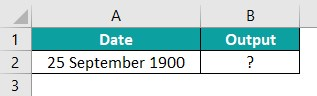Select cell B2, enter the formula =YEAR(A2)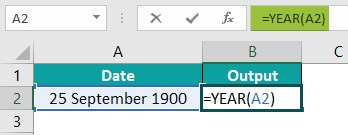Press the “Enter” key. The result is “1900”, as shown below.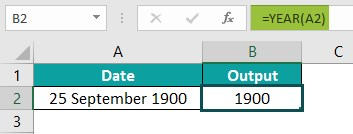3. Where is the YEAR function in Excel?

The YEAR function in Excel is in the “Formulas” tab and can be inserted as follows:

First, choose an empty cell – select the “Formulas” tab – go to the “Function Library” group – click the “Date & Time” option drop-down – select the “YEAR” function, as shown below.